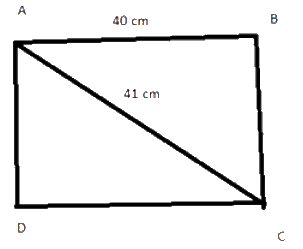# Ex.6.5 Q7 The Triangle and Its Properties - NCERT Maths Class 7

Go back to  'Ex.6.5'

## Question

Find the perimeter of the rectangle whose length is $$\rm{}40\, cm$$ and diagonal is $$\rm{}41\,cm$$.

Video Solution
Triangle & Its Properties
Ex 6.5 | Question 7

## Text Solution

What is known?

Length of rectangle is given $$\rm{}40\, cm$$ and diagonal is $$\rm{}41\,cm.$$

What is unknown?

Reasoning:

This question is based on the two concepts i.e. the concept of rectangle and Pythagoras theorem. For better understanding of this question understand it with the help of a figure.

As it is mentioned in the question suppose there is a rectangle $$ABCD$$ and whose length is given $$\rm{}40\,cm.$$ As, we know the opposite sides of a rectangle are equal if $$AB = \rm{}40$$$$\rm\,cm$$ that means side opposite to $$AB$$ i.e. $$CD$$ will also be $$\rm{}40\, cm.$$ Now, one of the diagonals of a rectangle is given $$AC = 41\,\rm\,cm$$ it divides the rectangle into two right-angled triangles. Now, you can apply Pythagoras theorem and find the third side i.e. breadth of the rectangle. Now, you have the measure of both the sides of a rectangle i.e. length and breadth, you can easily find out the perimeter of the rectangle.Steps:

Given,in rectangle $$ABCD,AB$$ and $$CD$$ are the lengths of the rectangle.

$$AB =\rm{}40\,cm,$$ $$CD = \rm{}40\,cm$$ and $$AC$$ is the diagonal

Therefore, $$AC = \rm{}41\,cm$$

Let breadth of rectangle be $$x$$.

Now, in triangle $$ADC,$$ by Pythagoras theorem

\begin{align}&\left( \text{Hypotenuse} \right)^2 \\&= \rm{{ }}{{\left(\text {Perpendicular} \right)}^2} + {\rm{ }}{{\left( \text{Base} \right)}^2} \end{align}

\begin{align} {{\left( {AC} \right)}^2} &= {{\left( {DC} \right)}^2} + {{\left( {AD} \right)}^2}\\{{\left( {41} \right)}^2} &= {{\left( {40} \right)}^2} +{{\left( x \right)}^2}\\1681 &= 1600{\rm{ }} + {{\left( x \right)}^2}\\1681-1600 &= {{\left( x \right)}^2}\\{x^2} &= 81\\{\rm{or}} \,x &= \rm{{ }}9{\rm{ }}cm\end{align}

Therefore, breadth of rectangle $$= \rm{}9\,cm$$

Now, we know that

Perimeter of rectangle

\begin{align} &= \rm{{ }}2\left( {l{\rm{ }} + {\rm{ }}b} \right)\\&= {\rm{ }}2{\rm{ }}\left( {40{\rm{ }} + {\rm{ }}9} \right)\\& = {\rm{ }}2\left( {49} \right)\\& = {\rm{ }}98{\rm{ }}\rm\,cm\end{align}

Hence, the perimeter of rectangle is $$98\rm\,cm$$

Useful Tip:

Whenever you encounter problems of this kind, remember the Pythagoras property of right -angled triangle and the formulas related to rectangle.

Learn from the best math teachers and top your exams

• Live one on one classroom and doubt clearing
• Practice worksheets in and after class for conceptual clarity
• Personalized curriculum to keep up with school